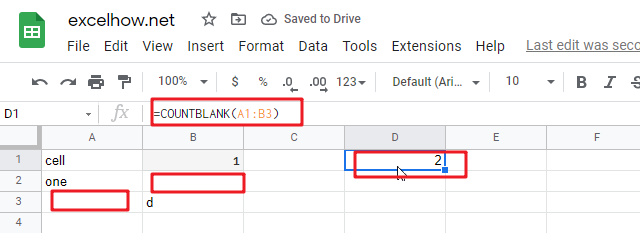# ExcelHow

This post will guide you how to use Google Sheets COUNTBLANK function with syntax and examples.

## Description

The Google Sheets COUNTBLANK function use to count the number of empty cells in a range of cells. You can use the COUNTBLANK function to get the number of blank cells in the given range in google sheets. This function will not count the cells that contain text, numbers, error.

The purpose of this function is used to count cells that are blank in google sheet and it’s returned value is a number of representing blank cells.

The COUNTBLANK function is a build-in function in Google Sheets and it is categorized as a MATH function.

## Syntax

The syntax of the COUNTBLANK function is as below:

= COUNTBLANK (range)

Where the COUNTBLANK function argument is:

• Range – This is a required argument.  The range from which you wish to count the empty cells.

Note:

• If one formula that return empty text, and it can be considered blank cell and it will be counted by the COUNTBLANK function.
• If cells contain zero and it will not be considered blank cell, so it will not be counted.

## Google Sheets COUNTBLANK Function Examples

The below examples will show you how to use google sheets COUNTBLANK Function to return the number of blank cells in a supplied range of cells.

1# to count empty cells in a given range A1:B3, using the following formula:

=COUNTBLANK(A1:B3)